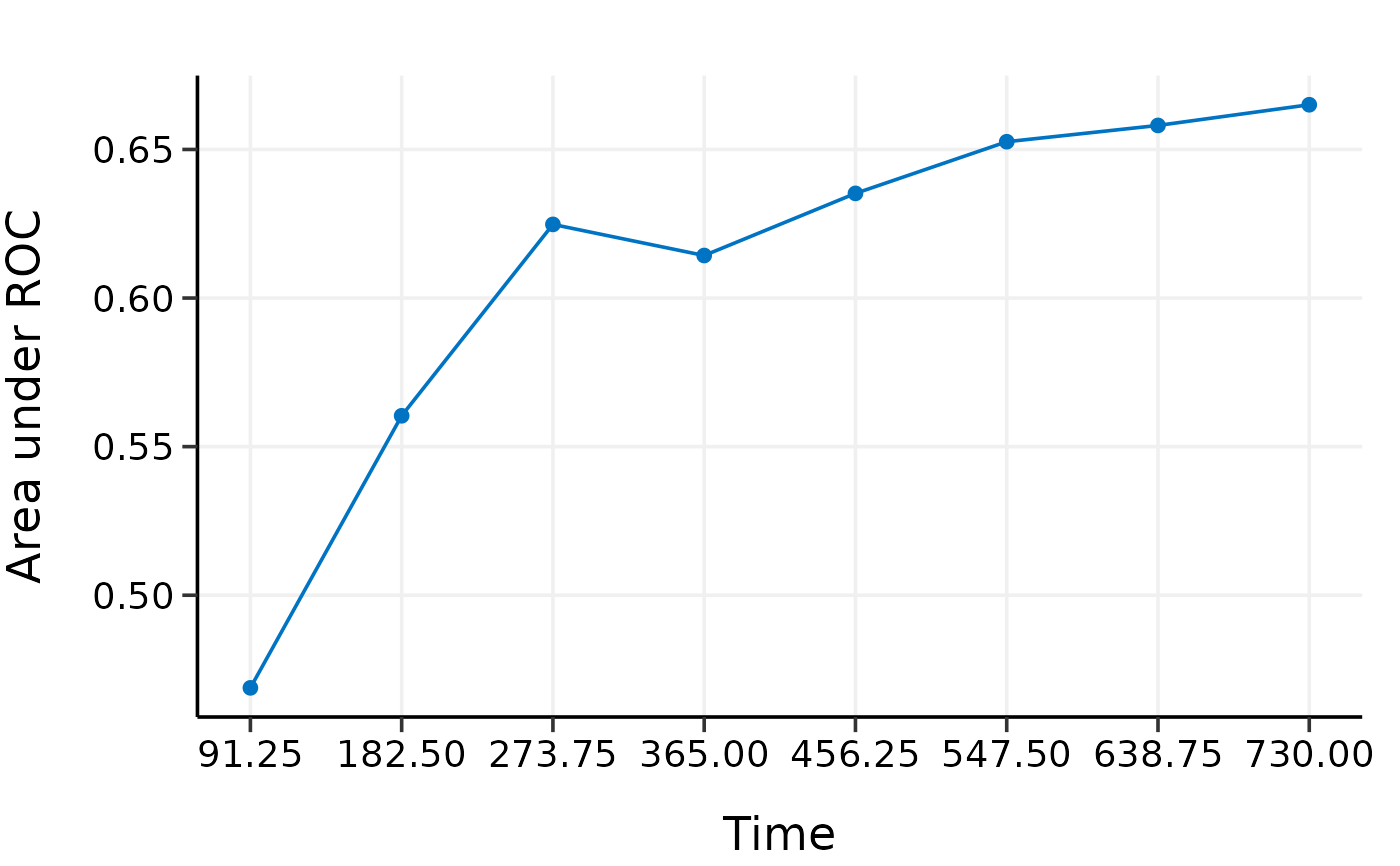Externally validate high-dimensional Cox models with time-dependent AUC

validate_external(object, x, time, event, x_new, time_new, event_new,
tauc.type = c("CD", "SZ", "UNO"), tauc.time)

## Arguments

object Model object fitted by hdnom::fit_*(). Matrix of training data used for fitting the model. Survival time of the training data. Must be of the same length with the number of rows as x. Status indicator of the training data, normally 0 = alive, 1 = dead. Must be of the same length with the number of rows as x. Matrix of predictors for the external validation data. Survival time of the external validation data. Must be of the same length with the number of rows as x_new. Status indicator of the external validation data, normally 0 = alive, 1 = dead. Must be of the same length with the number of rows as x_new. Type of time-dependent AUC. Including "CD" proposed by Chambless and Diao (2006)., "SZ" proposed by Song and Zhou (2008)., "UNO" proposed by Uno et al. (2007). Numeric vector. Time points at which to evaluate the time-dependent AUC.

Chambless, L. E. and G. Diao (2006). Estimation of time-dependent area under the ROC curve for long-term risk prediction. Statistics in Medicine 25, 3474--3486.

Song, X. and X.-H. Zhou (2008). A semiparametric approach for the covariate specific ROC curve with survival outcome. Statistica Sinica 18, 947--965.

Uno, H., T. Cai, L. Tian, and L. J. Wei (2007). Evaluating prediction rules for t-year survivors with censored regression models. Journal of the American Statistical Association 102, 527--537.

## Examples

data(smart)
# Use the first 1000 samples as training data
# (the data used for internal validation)
x <- as.matrix(smart[, -c(1, 2)])[1:1000, ]
time <- smart$TEVENT[1:1000] event <- smart$EVENT[1:1000]

# Take the next 1000 samples as external validation data
# In practice, usually use data collected in other studies
x_new <- as.matrix(smart[, -c(1, 2)])[1001:2000, ]
time_new <- smart$TEVENT[1001:2000] event_new <- smart$EVENT[1001:2000]

# Fit Cox model with lasso penalty
fit <- fit_lasso(
x, survival::Surv(time, event),
nfolds = 5, rule = "lambda.1se", seed = 11
)

# External validation with time-dependent AUC
val.ext <- validate_external(
fit, x, time, event,
x_new, time_new, event_new,
tauc.type = "UNO",
tauc.time = seq(0.25, 2, 0.25) * 365
)

print(val.ext)#> High-Dimensional Cox Model External Validation Object
#> Model type: lasso
#> Time-dependent AUC type: UNO
#> Evaluation time points for tAUC: 91.25 182.5 273.75 365 456.25 547.5 638.75 730summary(val.ext)#> Time-Dependent AUC Summary at Evaluation Time Points
#>         91.25     182.5    273.75       365   456.25     547.5    638.75
#> AUC 0.5146531 0.5771193 0.6329524 0.6168784 0.636541 0.6482618 0.6526306
#>           730
#> AUC 0.6576868plot(val.ext)#>         91.25     182.5    273.75       365   456.25     547.5    638.75
#> AUC 0.5146531 0.5771193 0.6329524 0.6168784 0.636541 0.6482618 0.6526306
#>           730
#> AUC 0.6576868# # Test fused lasso, MCP, and Snet models
# data(smart)
# # Use first 600 samples as training data
# # (the data used for internal validation)
# x <- as.matrix(smart[, -c(1, 2)])[1:600, ]
# time <- smart$TEVENT[1:600] # event <- smart$EVENT[1:600]
#
# # Take 500 samples as external validation data.
# # In practice, usually use data collected in other studies.
# x_new <- as.matrix(smart[, -c(1, 2)])[1001:1500, ]
# time_new <- smart$TEVENT[1001:1500] # event_new <- smart$EVENT[1001:1500]
#
# flassofit <- fit_flasso(x, survival::Surv(time, event), nfolds = 5, seed = 11)
# scadfit <- fit_mcp(x, survival::Surv(time, event), nfolds = 5, seed = 11)
# mnetfit <- fit_snet(x, survival::Surv(time, event), nfolds = 5, seed = 11)
#
# val.ext1 <- validate_external(
#   flassofit, x, time, event,
#   x_new, time_new, event_new,
#   tauc.type = "UNO",
#   tauc.time = seq(0.25, 2, 0.25) * 365)
#
# val.ext2 <- validate_external(
#   scadfit, x, time, event,
#   x_new, time_new, event_new,
#   tauc.type = "CD",
#   tauc.time = seq(0.25, 2, 0.25) * 365)
#
# val.ext3 <- validate_external(
#   mnetfit, x, time, event,
#   x_new, time_new, event_new,
#   tauc.type = "SZ",
#   tauc.time = seq(0.25, 2, 0.25) * 365)
#
# print(val.ext1)
# summary(val.ext1)
# plot(val.ext1)
#
# print(val.ext2)
# summary(val.ext2)
# plot(val.ext2)
#
# print(val.ext3)
# summary(val.ext3)
# plot(val.ext3)